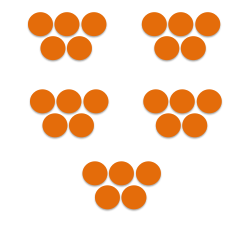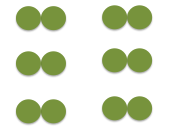Numbers 1-100: Lesson for Kids Video

Instructions:

Choose an answer and hit 'next'. You will receive your score and answers at the end.

question 1 of 3

Which of the following shows the correct way to count by 1s?

Create Your Account To Take This Quiz

As a member, you'll also get unlimited access to over 79,000 lessons in math, English, science, history, and more. Plus, get practice tests, quizzes, and personalized coaching to help you succeed.

Try it risk-free for 30 days. Cancel anytime

1. Which of the following shows the correct way to count the circles by 5s?2. Which of the following shows the correct way to count the circles by 2s?Create your account to access this entire worksheet
A Premium account gives you access to all lesson, practice exams, quizzes & worksheets
Quizzes, practice exams & worksheets
Certificate of Completion
Create an account to get started

How can you count numbers between 1-100? You will need to be familiar with different methods of counting to correctly answer the questions you will find in this quiz and worksheet.

Quiz & Worksheet Goals

For this quiz, you'll need to know how to:

• Count by 1's, 2's, 5's and 10's
• Apply one of these methods when counting circles given in an example

Skills Practiced

• Reading comprehension - make sure you understand the most important information from the lesson, such as using different counting methods
• Problem solving - use what you know to count the number of circles shown in a picture
• Information recall - remember how to count by 1's, 2's, 5's and 10's

You can expect to learn more in the lesson titled Numbers 1-100: Lesson for Kids. Use the lesson to find out:

• What kinds of things can be counted
• What a hundreds board is and what it looks like
• The definition of skip counting
Final ExamMath for Kids
Status: Not Started
Chapter ExamNumbers for Elementary School
Status: Not Started

Support